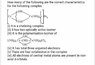# Coordination chemistry problem

• Chemistry
Amitkumarr
Homework Statement:
How many of the following are the correct characteristics for the following complex(see attached figure).

(i) It is a chelating complex
(ii) It has two optically active isomer
(iii) It is the polymerisation isomer of(see attached figure).
(iv) It has total three unpaired electrons (v) There are four octahedron in the complex
(vi) All electrons of central metal atoms are present in non axial d-orbitals.
Relevant Equations:
No relevant equations.Based on theory.
My attempt-
1)The given ligand forms chelate rings,so it should be a chelating complex.
2)It has no plane of symmetry (P.O.S),so it is optically active(octahedral complex) and should have 2 optically active isomers.
3)They are not polymerisation isomers because they have different emperical formula.
5) According to me there should be 3 octahedron in the complex.
For options 4 and 6,I am unable to get the oxidation state of Cr(central atom) and the overall charge of this ligand.What should be the contribution of the OH groups in the charge of this ligand?
Also,are my justifications correct?

#### Attachments

•IMG-20200708-WA0004.jpg
59.9 KB · Views: 115

Homework Helper
1, 2 - I agree.
3 - Do they have different empirical formulas? What are the EFs? (I assume we're talking about the complex ion, so the difference between Br and Cl doesn't count.)
5 - Only 3?
4, 6 - Count the OHs as OH- and the ammonias as neutral.

•etotheipi
Amitkumarr
1, 2 - I agree.
3 - Do they have different empirical formulas? What are the EFs? (I assume we're talking about the complex ion, so the difference between Br and Cl doesn't count.)
5 - Only 3?
4, 6 - Count the OHs as OH- and the ammonias as neutral.
3-They have the same emperical formula(if we don't consider Br and cl),so they are polymerisation isomers,right?
5-I think there should be 4 octahedron,1 on central atom and 3 on side atom.
If we count one OH as OH^- then we have 3×(-2)=-6 as overall charge of this ligand.So,the the oxidation state of Cr(central atom) would be x+(-6)=+6 which means x=+12 ,but that's not possible.
Where am I going wrong?
Note: Solution says only 3 of above options are correct.Which are wrong ones?

Homework Helper
x = +12, but there are four Cr atoms to divide this charge between.

•etotheipi and Amitkumarr
Amitkumarr
x = +12, but there are four Cr atoms to divide this charge between.
Thanks,I was missing that part.So, each Cr atom is in +3 O.S.
But,I have one more query about the first option.Can any ligand form chelate rings and simultaneously behave as bridging ligand(like in the above question)?